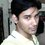# AprblmInTrigo Soln:

The Problem: AprblmInTrigo

$$\sin\alpha \sec ^{ 2 }{ \frac{\alpha }{2} } =l ...(A)$$ and $$\left( 1+\tan { \frac{\alpha }{2} } -\sec { \frac{\alpha }{2} } \right) \left( 1+\tan { \frac{\alpha }{2} } +\sec { \frac{\alpha }{2} } \right) =Cl ...(B)$$

The question simply asks for expressing (B) in terms of (A).\ i.e in terms like $\cos { \theta }$ and $\sin { \theta }$ . Since we now know our direction, lets sail mate.

$\left( 1+\tan { \frac{\alpha }{2} } -\sec { \frac{\alpha }{2} } \right) \left( 1+\tan { \alpha } +\sec { \frac{\alpha }{2} } \right) ={ \left( 1+\tan { \frac{\alpha }{2} } \right) }^{ 2 }-\sec ^{ 2 }{ \frac{\alpha }{2} } \\ =1+\tan ^{ 2 }{ \frac{\alpha}{2} } +2\tan { \alpha } /2-\sec ^{ 2 }{ \alpha } /2\\ =\left[ \cos ^{ 2 }{ \frac{\alpha}{2} } +\tan ^{ 2 }{ \frac{\alpha}{2} } \cos ^{ 2 }{ \frac{\alpha}{2} } +2\tan { \frac{\alpha}{2} } \cos ^{ 2 }{ \frac{\alpha}{2} } -1 \right] /\left[ \cos ^{ 2 }{ \frac{\alpha}{2} } \right] \\ =\left[ \left( \cos ^{ 2 }{ \frac{\alpha}{2} } +\sin ^{ 2 }{ \frac{\alpha}{2} } \right) +2\sin { \frac{\alpha}{2} } \cos { \frac{\alpha}{2} } -1 \right] /\left[ \cos ^{ 2 }{ \frac{\alpha}{2} } \right] \\= \left[ 2\sin { \frac{\alpha}{2} } \cos { \frac{\alpha}{2} } \right] /\left[ \cos ^{ 2 }{ \frac{\alpha}{2} } \right] =\sin { \alpha \sec ^{ 2 }{ \frac{\alpha}{2} } } =l$

Hence the answer is $1$Note by Soumo Mukherjee
6 years, 9 months ago

This discussion board is a place to discuss our Daily Challenges and the math and science related to those challenges. Explanations are more than just a solution — they should explain the steps and thinking strategies that you used to obtain the solution. Comments should further the discussion of math and science.

When posting on Brilliant:

• Use the emojis to react to an explanation, whether you're congratulating a job well done , or just really confused .
• Ask specific questions about the challenge or the steps in somebody's explanation. Well-posed questions can add a lot to the discussion, but posting "I don't understand!" doesn't help anyone.
• Try to contribute something new to the discussion, whether it is an extension, generalization or other idea related to the challenge.

MarkdownAppears as
*italics* or _italics_ italics
**bold** or __bold__ bold
- bulleted- list
• bulleted
• list
1. numbered2. list
1. numbered
2. list
Note: you must add a full line of space before and after lists for them to show up correctly
paragraph 1paragraph 2

paragraph 1

paragraph 2

[example link](https://brilliant.org)example link
> This is a quote
This is a quote
    # I indented these lines
# 4 spaces, and now they show
# up as a code block.

print "hello world"
# I indented these lines
# 4 spaces, and now they show
# up as a code block.

print "hello world"
MathAppears as
Remember to wrap math in $$ ... $$ or $ ... $ to ensure proper formatting.
2 \times 3 $2 \times 3$
2^{34} $2^{34}$
a_{i-1} $a_{i-1}$
\frac{2}{3} $\frac{2}{3}$
\sqrt{2} $\sqrt{2}$
\sum_{i=1}^3 $\sum_{i=1}^3$
\sin \theta $\sin \theta$
\boxed{123} $\boxed{123}$

Sort by:

Please don't use so weird formatting. Only give the \ ( .... \ ) wrapping around the Math Terms and not around the words.

- 6 years, 9 months ago

Thanx buddy! Nxt time I will.

- 6 years, 9 months ago

I've edited this, take a note of the changes and use what you understand in future.

- 6 years, 9 months ago

yep.thanx again.

- 6 years, 9 months ago